• capacitymax_size ...vector<int> a; cout << "capacity: " << a.capacity() << endl; cout << "max_size" << a.max_size() << endl; cout << "size: " <&...

capacity  max_size

vector<int> a;
cout << "capacity: " << a.capacity() << endl;
cout << "max_size" << a.max_size() << endl;
cout <<	"size: " << a.size() << endl;

capacity: 0
str capacity: 153391689
max_size：1073741823
size: 0

STL容器的capacity属性，表示STL在发生realloc前能允许的最大元素数，也可以理解为预分配的内存空间。例如一个vector<int> v的capacity为5，当插入第6个元素时，vector会realloc，vector内部数据会复制到另外一个内存区域。这样之前指向vector中的元素的指针、迭代器等等均会失效。
max_size属性和capacity不同，表示STL容器允许的最大元素数，通常，这个数是一个很大的常整数，可以理解为无穷大。这个数目与平台和实现相关，在我的机器上vector<int> 、<string>分别如上图。因为max_size很大，所以基本不会发生元素数超过max_size的情况，只需知道两者区别即可。

capacity size

size是当前vector容器真实占用的大小，也就是容器当前拥有多少个容器。

capacity是指在发生realloc前能允许的最大元素数，即预分配的内存空间。

这两个属性分别对应两个方法：resize()和reserve()。

使用resize()，容器内的对象内存空间是真正存在的。

使用reserve()仅仅只是修改了capacity的值，容器内的对象并没有真实的内存空间(空间是"野"的)，此时切记使用[]操作符访问容器内的对象，很可能出现数组越界的问题。

vector<int> v; //此时没有初始化，所以size()和capacity()都是0；
cout << v.size() << "; "  << v.capacity() << endl;
v.push_back(1);
cout << v.size() << "; "  << v.capacity() << endl;//容器内有一个元素，那么size和capacity都是1
v.push_back(1);
cout << v.size() << "; "  << v.capacity() << endl;//容器内capacity()空间不够，需要申请内容，此时有两个元素，size为2，capacity是2
v.push_back(1);
cout << v.size() << "; "  << v.capacity() << endl;//此时容器能够提供的空间是2，又增加元素，不够，需要申请空间，size为3，capacity为4
v.push_back(1);
cout << v.size() << "; "  << v.capacity() << endl;//容器还能够提供一个空间，不需要申请新空间，size为4，capacity为4
v.push_back(1);
cout << v.size() << "; "  << v.capacity() << endl;//空间不够，需要申请，size()为5，capacity为6

vector::reserve
函数原型：
void reserve(size_type n);
reserve是容器预留空间，但并不是真正创建元素。在创建对象前，不能引用容器中的元素，当插入元素时，需要用push_back()和insert()。
reserve有一个参数：n为预留空间的大小。

vector::resize
函数原型：
void resize(size_type sz,T c = T());
resize是改变容器的大小，并创建对象。调用此函数时，可以引用容器中的对象，当加入新元素时，可以调用operator[]。
resize有两个参数：第一个参数为容器的新大小；第二个参数为加入容器的新元素。第二个参数可缺省。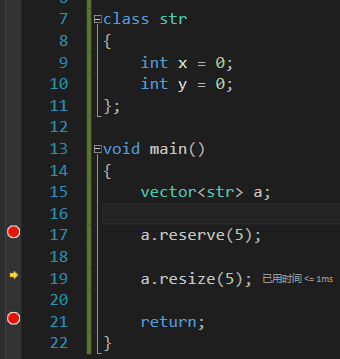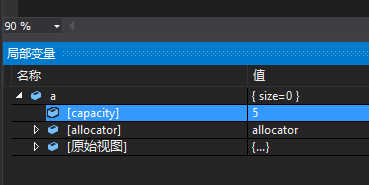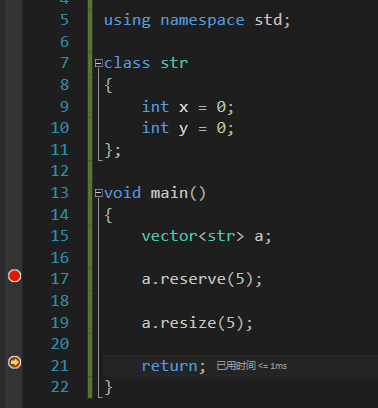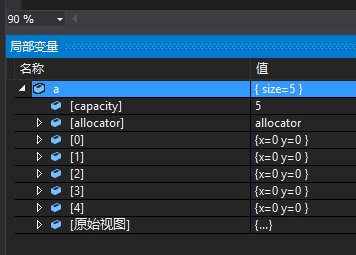代码中，在变量s，被resize为10后，其内容如下，可以清晰的看到，其resize并不会改变vector中已经存在的值，只会将重新申请出来的栈空间内的值变为0。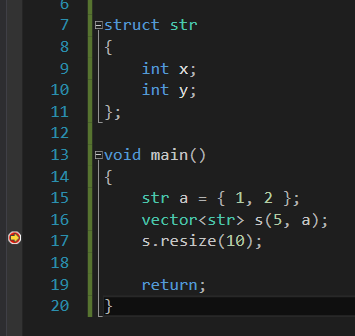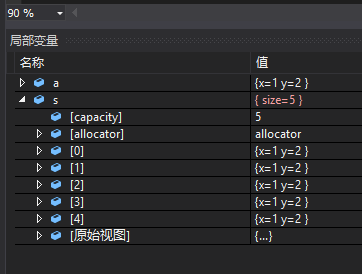代码中，在变量s，被resize为5后，其内容如下，可以清晰的看到，其resize并不会改变vector中已经存在的值。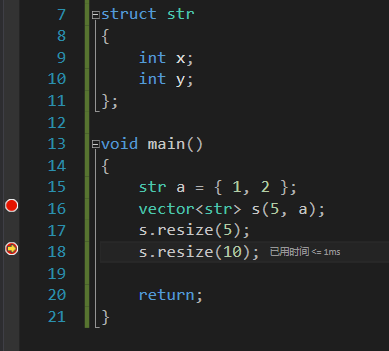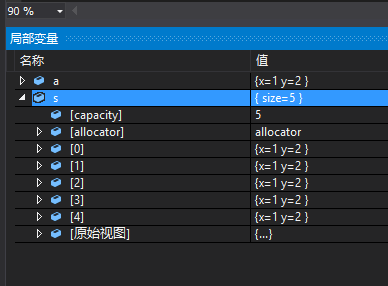代码中，在变量s，被resize为10后，其内容如下，可以清晰的看到，其resize并不会改变vector中已经存在的值，只会将重新申请出来的栈空间内的值变为0。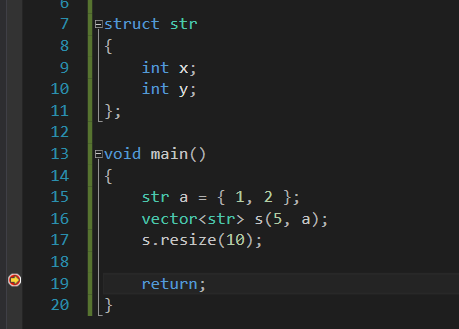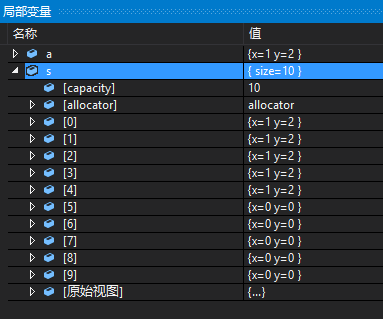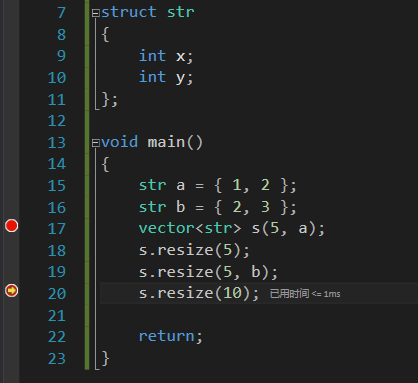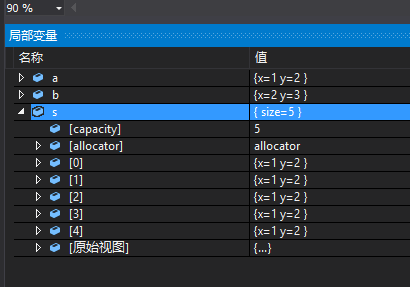resize不能用作重新初始化vector，那用什么方法比较好呢？其实可以直接定义一个初始化vector，如原vector的size相同，其值为初始化值。而vector之间的赋值，为深拷贝，这样就能够直接对vector的值进行初始化了，且效率很高（如代码）。

参考：

https://blog.csdn.net/jinx_qixianzhi/article/details/83143748

展开全文• vector size的使用 0、vectorsize函数的返回值是一个无符号类型，当size是0的时候，对其进行–，会造成数字的越界溢出 1、size是指向vector中数组长度后的那一个位置，即size处没有元素 2、vector的下标是从零...
vector size的使用
0、vector中size函数的返回值是一个无符号类型，当size是0的时候，对其进行–，会造成数字的越界溢出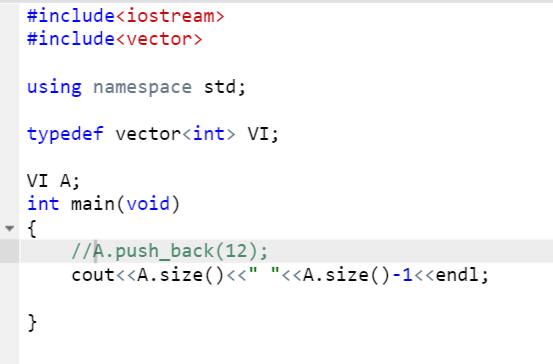1、size是指向vector中数组长度后的那一个位置，即size处没有元素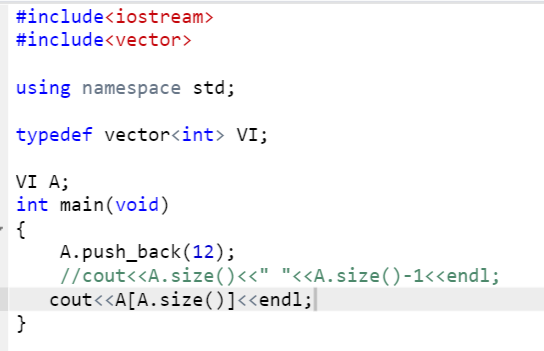2、vector的下标是从零开始的；


展开全文c++ 数据结构
• // vector::size #include <iostream> #include <vector> using namespace std; //返回的大小 //返回向量中元素的个数。 //这是向量中实际持有的对象的数量，这并不一定等于它的存储容量。 int main ...
// vector::size
#include <iostream>
#include <vector>

using namespace std;

//返回的大小
//返回向量中元素的个数。
//这是向量中实际持有的对象的数量，这并不一定等于它的存储容量。

int main ()
{
vector<int> myints;
cout << "0. size: " << myints.size() << '\n';

for (int i=0; i<10; i++) myints.push_back(i);
cout << "1. size: " << myints.size() << '\n';

myints.insert (myints.end(),10,100);
cout << "2. size: " << myints.size() << '\n';

for(vector<int>::iterator it=myints.begin();it!=myints.end();++it)
{
cout<<*it<<" ";
}
cout<<endl;

myints.pop_back();
cout << "3. size: " << myints.size() << '\n';

return 0;
}

/*
Output:
0. size: 0
1. size: 10
2. size: 20
3. size: 19
*/



展开全文• 首先看一段代码： vector<int> a{1,2,3}; cout << a.size() ; vector<int> b{}; cout << b.size() ; cout << a.size() - 3 ; cout << b.size() - 3 ;程序运行结果： 可以发现 b.size
首先看一段代码：

    vector<int> a{1,2,3};
cout << a.size() << endl;
vector<int> b{};
cout << b.size() << endl;

cout << a.size() - 3 << endl;
cout << b.size() - 3 << endl;

程序运行结果：可以发现 b.size() - 3处仍然大于0，属于unsigned类型，以后在判断时利用int类型判断时应注意。
展开全文• vector::insert // vector::get_allocator #include #include using namespace std; int main () { vector myvector (3,100); //原始 vector::iterator it; it = myvector.begin(); it = myvector.insinsert operator
• java vector 矢量类size()方法 (Vector Class size() method) size() method is available in java.util package. size()方法在java.util包中可用。 size() method is used to return the size (i.e. the number ...java python redis 多线程 jvm
• java setsize 向量类setSize()方法 (Vector Class setSize() method) setSize() method is available in java.util package. setSize()方法在java.util包中可用。 setSize() method is used to set the new size of ...java python redis jvm spring
• vector.size(),返回向量中元素个数 vector.capacity()，返回向量的容量2. 当添加元素时，超过了容器的容量(即capacity)，则将从新分配容器内存空间，迭代器也会失效，迭代器需要重新赋值 每次增加原容量的一半#...
• ## C++ vector.size()

万次阅读 2019-05-20 09:33:25
vectorsize函数返回vector大小，返回值类型为size_type，Member type size_type is an unsigned integral type，即无符号整数； vector A; A.size()-1因为size返回值是无符号类型所以 A.size()-1越界，是个很大的...
• 正确使用vector.size-1,应该是符号问题。
• vectorsize是一个函数，因此要写成 .size() Mat的size是一个成员变量，写法是 .size
• vectorsize函数返回vector大小，返回值类型为size_type，Member typesize_typeis an unsigned integral type，即无符号整数； vector<int> A; A.size()-1因为size返回值是无符号类型所以 A.size()-1越界...
• //////////////////////////////////////// ...// vector-max_size #include &lt;iostream&gt; #include &lt;vector&gt; using namespace std; int main(){ vector&lt;int&gt; ...
• ## vector的capacity和size

千次阅读 2012-10-28 11:22:02
vector提供了capacity和size获取大小。但是这两个大小是不一样的。有结论：capacity一定是大于或者等于size的。 解释如下： 当我们使用 vector vec(10,9) 创建一个vector时，实际上是执行了以下函数： void...
• 问题On 32 bit System.std::vector::max_size() returns 232-1, size of char — 1 bytestd::vector::max_size() returns 230-1, size of int — 4 bytestd::vector::max_size() returns 229-1, size of double — 8...
• 主要介绍了vector 容器大小相关的操作函数（size、max_szie、capacity），同时介绍了容器大小超出容器capacity能力会内存重新分配以及iterator失效简单分析。同时给出了避免内存重新分配的两种方法。capacity
• for( vector ::size_type j=vec.size()-1; j>=0; --j ) 会出现死循环for( int j=vec.size()-1; j>=0; --j ) 却是正确的。 size_type是unsigned int型的，当j==0之后，j--，那么j理应为-1，但是unsigned属性，...
• vector&lt;vector&lt;int&gt;&gt;b实例化一个名为b的vector。这个vector当中存得是一系列的vector&...(a.size())，即一个长度为a.size()，里面值都填充为0的vector&lt;int&gt;...
• 写代码时遇到vectorsize比较，代码片截取修改如下 #include<iostream> #include<vector> using namespace std; int main() { vector<int> A; A = { 2,-1,2 }; int x = A.size() - 4; if (0&...stl c++
• vector对象的sizevector类型总是包括vector的元素类型vector<int>::size_type //ok vector::size_type //errorvector的遍历1、下标遍历2、迭代器遍历end操作返回的迭代器指向vector的末尾元素的下一个，表明它指向一...迭代器
• Env: Mac OSX 10.9.2 c++11#include #include <vector>int main (int argc, char *argv[]) { int n = 5; std::vector<int> nv(n, -1); std::vector<int> nv1, nv2, nv3, nv4, nv5; nv1.c++
• f(leaves.size(),vector< int >(3) ) 的解释 问题解释： vector<vector< int > >f：定义一个二维迭代器，一层迭代器里面放着另一层迭代器，相当于动态二维数组； vector<vector< int> ...数据结构 c++
• stl vector 函数 C ++ vector :: size()函数 (C++ vector::size() function) vector::size() is a library function of "vector" header, it is used to get the size of a vector, it returns the total number of ...c++ python javascript java 深度学习
• vector中的size（）函数返回当前数组的元素数量，push_back进去几个就返回几个。 capacity是返回数组的大小，跟插入的数量有关，vector的容量size是比其数组size第一个大的二进制数。 即可能的capacity值为 1 2 ...
• 总共放入a.size()个默认值，默认值的内容是vector(a.size())，即一个长度为a.size()，里面值都填充为0的vector。 可以把b想象成一个表，它有a.size()行，其中每行又有a.size()列。新建的表里面这a.sic++ STL
• 原文地址：http://www.cplusplus.com/reference/vector/vector/max_size/public member function&lt;vector&gt;std::vector::max_sizeC++98C++11size_type max_size() const noexcept;Return maximum size...
• 后， vector对象a的size和capacity都是0。 当插入第一个元素后，size和capacity都变为1 当插入第二个元素后，size和capacity都变为2 当插入第三个元素后，size变为3， 由于capacity为2，小于3， 则capacity=......